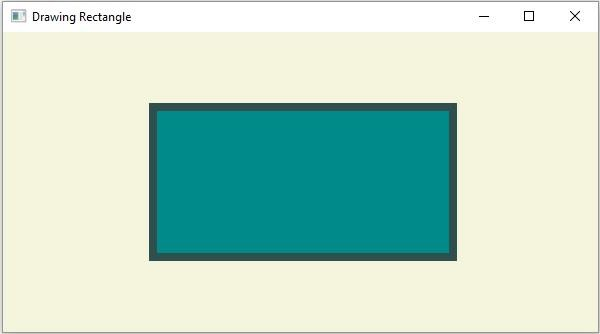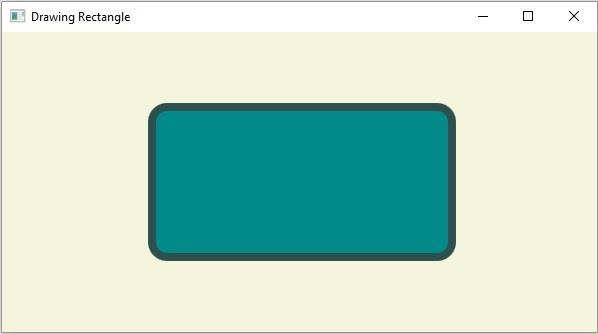# How to create a Rectangle using JavaFX?

A Rectangle is a closed a polygon with four edges, the angle between any two edges is a right angle and the opposite sides are concurrent. It is defined by its height and width, the lengths of the vertical and horizontal sides respectively.

In JavaFX a Rectangle is represented by the javafx.scene.shape.Rectangle class. This class contains four properties they are −

• height − This property represents the x coordinate of the center of a circle, you can set the value to this property using the setHeight() method.

• width − This property represents y coordinate of the center of a circle, you can set the value to this property using the setWidth() method.

• x − The radius of the circle in pixels, you can set the value to this property using the setRadius() method.

• y − The radius of the circle in pixels, you can set the value to this property using the setRadius() method

To create a Rectangle you need to −

• Instantiate the class Rectangle.

• Set the required properties using the setter methods or, bypassing them as arguments to the constructor.

• Add the created node (shape) to the Group object.

## Example

import javafx.application.Application;
import javafx.scene.Group;
import javafx.scene.Scene;
import javafx.scene.paint.Color;
import javafx.stage.Stage;
import javafx.scene.shape.Rectangle;
public class DrawinRectangle extends Application {
public void start(Stage stage) {
//Drawing a Rectangle
Rectangle shape = new Rectangle();
//Setting the properties of the rectangle
shape.setX(150.0f);
shape.setY(75.0f);
shape.setWidth(300.0f);
shape.setHeight(150.0f);
//Setting other properties
shape.setFill(Color.DARKCYAN);
shape.setStrokeWidth(8.0);
shape.setStroke(Color.DARKSLATEGREY);
//Setting the Scene
Group root = new Group(shape);
Scene scene = new Scene(root, 595, 300, Color.BEIGE);
stage.setTitle("Drawing Rectangle");
stage.setScene(scene);
stage.show();
}
public static void main(String args[]){
launch(args);
}
}

## Output## Rounded Rectangle

In addition to the above-mentioned properties. The Rectangle class also provides two more properties namely −

• arcWidth − This property represents the diameter of the arc at the 4 corners. You can set value it using the setArcWidth() method.

• arcHeight − This property represents the height of the arc at the 4 corners. You can set value it using the setArcHeight() method.

By setting values to these you can draw a rectangle with rounded/arced edges −

## Example

import javafx.application.Application;
import javafx.scene.Group;
import javafx.scene.Scene;
import javafx.scene.paint.Color;
import javafx.stage.Stage;
import javafx.scene.shape.Rectangle;
public class DrawingRoundedRectangle extends Application {
public void start(Stage stage) {
//Drawing a Rectangle
Rectangle shape = new Rectangle();
//Setting the properties of the rectangle
shape.setX(150.0f);
shape.setY(75.0f);
shape.setWidth(300.0f);
shape.setHeight(150.0f);
shape.setArcHeight(30.0);
shape.setArcWidth(30.0);
//Setting other properties
shape.setFill(Color.DARKCYAN);
shape.setStrokeWidth(8.0);
shape.setStroke(Color.DARKSLATEGREY);
//Setting the Scene
Group root = new Group(shape);
Scene scene = new Scene(root, 595, 300, Color.BEIGE);
stage.setTitle("Drawing Rectangle");
stage.setScene(scene);
stage.show();
}
public static void main(String args[]){
launch(args);
}
}

## Output